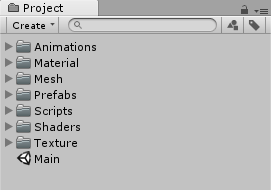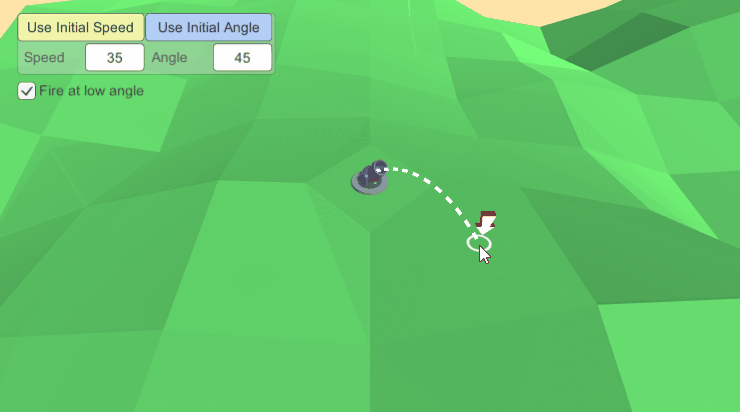# はじめに

「ProjectileShooting」は放物線を描いて弾を発射するサンプルです

# デモ「Main」シーンを開いて Unity を再生することで動作確認できます# 実装方法

「ProjectileMath.cs」に記載されています

```using UnityEngine;

public static class ProjectileMath
{
/// <summary>
/// Calculates the two possible initial angles that could be used to fire a projectile at the supplied
/// speed to travel the desired distance
/// </summary>
/// <param name="speed">Initial speed of the projectile</param>
/// <param name="distance">Distance along the horizontal axis the projectile will travel</param>
/// <param name="yOffset">Elevation of the target with respect to the initial fire position</param>
/// <param name="gravity">Downward acceleration in m/s^2</param>
/// <param name="angle0"></param>
/// <param name="angle1"></param>
/// <returns>False if the target is out of range</returns>
public static bool LaunchAngle(float speed, float distance, float yOffset, float gravity, out float angle0, out float angle1)
{
angle0 = angle1 = 0;

float speedSquared = speed * speed;

float operandA = Mathf.Pow(speed, 4);
float operandB = gravity * (gravity * (distance * distance) + (2 * yOffset * speedSquared));

// Target is not in range
if (operandB > operandA)
return false;

float root = Mathf.Sqrt(operandA - operandB);

angle0 = Mathf.Atan((speedSquared + root) / (gravity * distance));
angle1 = Mathf.Atan((speedSquared - root) / (gravity * distance));

return true;
}

/// <summary>
/// Calculates the initial launch speed required to hit a target at distance with elevation yOffset.
/// </summary>
/// <param name="distance">Planar distance from origin to the target</param>
/// <param name="yOffset">Elevation of the origin with respect to the target</param>
/// <param name="gravity">Downward acceleration in m/s^2</param>
/// <param name="angle">Initial launch angle in radians</param>
/// <returns>Initial launch speed</returns>
public static float LaunchSpeed(float distance, float yOffset, float gravity, float angle)
{
float speed = (distance * Mathf.Sqrt(gravity) * Mathf.Sqrt(1 / Mathf.Cos(angle))) / Mathf.Sqrt(2 * distance * Mathf.Sin(angle) + 2 * yOffset * Mathf.Cos(angle));

return speed;
}

/// <summary>
/// Samples a series of points along a projectile arc
/// </summary>
/// <param name="iterations">Number of points to sample</param>
/// <param name="speed">Initial speed of the projectile</param>
/// <param name="distance">Distance the projectile will travel along the horizontal axis</param>
/// <param name="gravity">Downward acceleration in m/s^2</param>
/// <param name="angle">Initial launch angle in radians</param>
/// <returns>Array of sampled points with the length of the supplied iterations</returns>
public static Vector2[] ProjectileArcPoints(int iterations, float speed, float distance, float gravity, float angle)
{
float iterationSize = distance / iterations;

Vector2[] points = new Vector2[iterations + 1];

for (int i = 0; i <= iterations; i++)
{
float x = iterationSize * i;
float t = x / (speed * Mathf.Cos(radians));
float y = -0.5f * gravity * (t * t) + speed * Mathf.Sin(radians) * t;

Vector2 p = new Vector2(x, y);

points[i] = p;
}

return points;
}
}
```